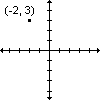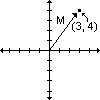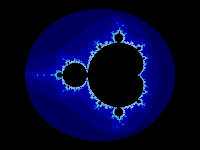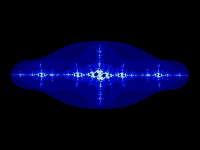• # Fractal Primer

## Introduction

Welcome to Judebert's How to Make a Mandelbrot. This is a tutorial on how to make fractal images on your computer, including Mandelbrot sets. I'll show you the math, include some source code fragments, and generally help you along. I assume you already know a programming language like C, but I'll refresh you on the math as we go.

To understand fractals, you must understand complex numbers. I've included a quick refresher course. To avoid boring anyone, you can skip over the stuff you already know, straight to the stuff you're looking for:

## Conventions

I don't want to include a lot of graphics, so I've got to set up some conventions.

Multiplication will be represented by the asterisk: \*. For instance, 3 \* 6 = 18.

When I want the square root of a number, I'll write sqrt(), like in C. For instance, sqrt(4) = 2.

When I want the square of a number, I'll write **2. For instance, 2**2 = 4. I'll also use this for other exponents, like cubing: 2**3 = 8.

I'll write examples on their own lines, to make them easier to follow.

## Complex Numbers

A complex number is a number including the square root of -1. If you remember your algebra, you know that any number sqared is positive; therefore no known number squared can result in -1, since it is negative. Since it doesn't exist, but we have to use it to solve some mathematical problems, we call it "imaginary." We have to use sqrt(-1) often enough that we've given it a special symbol, based on the fact that it's imaginary: i.

Since i is an imaginary number, we couldn't just subtract it from other numbers. What is 2 - i? Well, it's not 1. It's not 3. It's not any number we could actually name. Therefore we just call it (2 - i). Same thing goes for addition, multiplication, division, anything. You might notice that (2 - i) has more two parts: the real part, which is 2, and the imaginary part, which is i. Since this number contains more parts than a simple number, we call it a "complex" number.

Dealing with these numbers is not as difficult as it sounds. Consider trying to add two complex numbers. To complete this mind-bogglingly complex calculation, simply add the parts together. Like this:

```    (2 + i)
+(1 + i)
-- --------------
(3 + 2i)
```
That's all. This example gives you an glimpse at multiplication, too: just like for any other number, 2i is the same as i + i.

Note that you can add complex and simple numbers, too. This is easy because you can think of a simple number as a complex number with the i set to 0. For instance, 5 can be thought of as (5 + 0i). So to add (2 + i) and 5, you could do this:

```    (2 +  i)
+(5 + 0i)
----------------
(7 +  i)
```

Here's another example:

```    (2 + i)
+(1 - i)
---------------
(3 + 0i), which is 3.
```
Got it? Good. The same thing works for subtraction.

Now for multiplication. As you recall from above, i is sqrt(-1); therefore i**2, or i squared, is -1. This is simple, so I'll only say it one more time: since sqrt(x)**2 (the square root of x, squared) is x, i**2 is sqrt(-1)**2, which is -1. Thus ends the lesson in definitions and identities.

The tricky part is multiplying complex numbers. For instance, what is

```    (2 + i) \* (1 + 2i)?
```
Well, the thing to do here is to remember your algebra. This is the same thing as
```    (2 + x) \* (1 + 2x)
```
which you can multiply with the FOIL method. Certainly you remember FOIL. FOIL stands for First, Outer, Inner, Last -- the way you multiply polynomials. In this case:
```    (2 + x) \* (1 + 2x)
The First terms are 2 and 1.             2 \* 1  =  2.
The Outer terms are 2 (again) and 2x.    2 \* 2x = 4x.
The Inner terms are x and 1.             x \* 1  =  x.
The Last  terms are x and 2x.            x \* 2x = 2x**2.
```
So we wind up with 2 + 4x + x + 2x**2, which when added together make 2 + 5x + 2x**2. Curses, FOILed again! :)

Well, the original question was about multiplying two complex numbers; so we substitute i for the x and get 2 + 5i + 2i**2. And, as you recall from the start of the discussion, i**2 is -1, so the 2i**2 is best written as -2. That changes the polynomial a bit, to 2 + 5i + (-2), which is 0 + 5i, or just 5i, if you like.

If you got lost, go back and read it again. This is important to the whole idea of fractals.

One last note before moving on: there's a simpler way to see the outcome when you square a polynomial. Here it is, using no numbers, all letters:

```    (a + bx) \* (a + bx)
The First terms are a and a.             a \* a  = a**2
The Outer terms are a (again) and bx.    a \* bx = abx.
The Inner terms are bx and a.            bx \* a = abx.
The Last  terms are bx and bx.           bx \* bx = (b**2)(x**2)
```
So we wind up with a**2 + 2abx + (b**2)(x**2). With complex numbers, that x is actually i, so (x**2) is going to be (i**2), which is -1 (as you recall), so we actually wind up with a**2 + 2abi - b**2. It's best to write this with the real numbers on the left, and the imaginary numbers on the right, just like a complex number. The final formula is:

(a + bi)**2 = a**2 - b**2 + 2abi.

## The Complex Plane

The Mandelbrot uses a complex plane; all fractals do.You're accustomed to a number plane with x going left-to-right (horizontal) and y going up-and-down (vertical). (At least, I HOPE you're familiar with this idea.) The complex plane is a very simple modification on this standard number plane, with the vertical axis being imaginary numbers. That's right, instead of ...-2, -1, 0, 1, 2... the vertical axis is ...-2i, -1i, 0i, 1i, 2i...

That makes it easy to place complex numbers on the plane. For instance, (2 - i) could be drawn as a point at (2, -1). (-2 + 3i) could be drawn as a point at (-2, 3). This is going to come in handy in just a moment.Finally, the last idea about complex numbers and the complex plane you need to know: magnitude. This is just the distance from the origin of the complex plane to the point. You use the pythagorean theorem for this one: a**2 + b**2 = c**2. So, the complex number (3 + 4i), which lies at (3, 4) on the complex plane, has a magnitude of:

```
3**2 + 4**2 = magnitude**2
9 + 16 = magnitude**2
25 = magnitude**2
5 = magnitude
```
for a magnitude of 5.

## The Mandelbrot

You might notice that all those complex numbers have some interesting properties. For instance, Benoit Mandelbrot noticed that if you square the number, then add it on to your figure, and kept doing that forever, the number will either become infinitely large or it will tend to go towards 0. The formula looks like this:
```    z = z**2 + c
or more computerish
z = last_z**2 + c.
```
Consider (2, 0) for example. We start z and last_z at 0, and set c to (2 + 0i). Then:
```    0: last_z**2 + c =
0**2 + (2 + 0i), which is
(2 + 0i).
```
So now we set last_z to (2 + 0i) and try again. I'll use the simple formula for squaring complex numbers that we developed above:
```
1: last_z**2 + c =
(2 + 0i)**2 + c =
(4 - 0 + 0i) + c =
(4 + 0i) + (2 + 0i) =
(6 + 0i).
```
So now we set last_z to 6 and try again. It's easy to see that this is going to get really big really fast, so let's just leave it here and say that it's going to become infinitely large.

That was the easy example. For a harder one, consider (0, -1). We start z and last z at 0, then set c to (0 - i). Then:

```
0: last_z**2 + c =
0**2 + (0 - i) =
(0 - i).
```
In the future, you advanced mathematicians can just start with last_z = c, since that's the way it's always going to work out. Next step! Set last_z to (0 - i) and do it again:
```    1: last_z**2 + c =
(0 - i)**2 + c =
(0 - 1 + 0i) + c =
(-1 + 0i) + c =
(-1 + 0i) + (0 - i) =
(-1 - i)
```
So far so good. Let's try another iteration. Set last_z to (-1 - i) and:
```
2: last_z**2 + c =
(-1 - i)**2 + c =
(1 - 1 + 2i) + c =
(0 + 2i) + c =
(0 + 2i) + (0 - i) =
(0 + i)
```
Still no guess. We can't tell what's going to happen yet. I'll skip the math examples and just let you know what the next few iterations will give us:
```    3: (-1 - i)
4: ( 0 + i)
5: (-1 - i)
6: ( 0 + i)
7: (-1 - i)
```
Anyone see a pattern developing? This is going to keep up all day. As we iterate infinite times, this number goes towards 0.

Obviously it's nice to have a computer to do all this figuring for us. I'll leave the programming to you; come up with a nice representation of complex numbers and use it. Or get a fractal program that already does this stuff for you.

For these examples, we could tell intuitively whether they would become infinitely large. But the computer will need some rules. How can the computer tell if we're going infinite?

Some lucky mathematician already solved this problem for us. He proved that if the magnitude of a complex number was greater than 2, then it would go to infinity when used in Mandelbrot's formula. So if we ever get a number of magnitude greater than 2, we're done with that point. And it's easy to see that (-23, -8) has a magnitude much greater than 2, so it will go to infinity, just as we suspected. (Another note for the advanced mathematicians: why bother to take the square root? You get the square of the magnitude along the way; just check to see if its greater than 4.)This is where the pretty picture comes in. We can see the Mandelbrot set will never go farther up, down, left or right than 2, since (0, 2), (2, 0), (-2, 0), and (0, -2) all have magnitude greater than 2. So we start at (-2, 2) and work pixel-by-pixel towards (2, -2). If the number goes to infinity (magnitude > 2) we draw a point there and move on. If it doesn't, we draw a black point (or don't draw the point at all) and move on.

Wait... what about the ones that don't go to infinity?

Their magnitude never goes over 2, so if we only check for numbers getting too big we'll be stuck working complex multiplication all day. Computers are fast, but an endless loop is still an endless loop. You'll never get to infinity, no matter how fast you run.

So we'd better limit how long we're willing to work this problem. If after, say, 100 calculations your number still hasn't reached a magnitude of 2, we'll guess that it's on its way to 0 and draw it black.

This also gives us a nice way to add color to our picture.Some numbers start on their way to infinity faster than others. (2, 2) does it right off. If we look at how many times we had to calculate before we stop, we could color pixels according to how fast the number "escapes." The faster pixels could be closer to white (or blue, or whatever) while the slower ones get darker and darker. (Actually it looks better the other way, with faster pixels dark and slower pixels bright, but who cares? Color it however you like it.) The pixels that never escape we color black.

That gives you the classic Mandelbrot, right there on your screen. Cool, huh?

## Anything else?

There are a few things you should be careful about if you intend to take this any farther.

We've been working with integers; the Mandelbrot set we draw works with real numbers. Like (1.5768293 + 0.2836589i). Computers are great, aren't they? If you're drawing from (-2, 2) to (2, -2), then your number plane is 4.0 across. If you've got 640 pixels accross the screen, then you want to start at -2.0 and add (4.0 / 640) = 0.00625 each time until you get to 2. Get the idea? That's how all these examples were drawn.

Why just draw from (-2, 2) to (2, -2)? That shows you the whole set. It's pretty, but... there are more interesting things farther down. Try drawing from (-0.5, 2) to (0.5, 1.0). Or other places. Just be careful: some of those places that look black aren't really black. They're pixels that would have gone to infinity if we waited longer (they're escaping really slowly). Try 150, 1000, 1500, 10000 iterations. It'll take longer, but you'll get to see more of the Mandelbrot set, and in better detail, too.

If you try more iterations than you have colors, you could run into trouble. For instance, if you have a table of 256 colors, and you find a pixel that escapes after 300 iterations, what color do you paint it? Maybe you should try to map the iterations into a colormap differently. You could spread the iterations through the color bands. Or find a way to map into 24 bit color.

While we're on the subject, why use the iterations to color the pixels? I only mentioned it because it's simple and easy. You could use the magnitude of the pixel when it escaped as its color. Or you could use the iteration as the red component, the magnitude as the blue component, and the start number as the green. What the hell; it can't be worse than some of the stuff I've tried.Julia sets. A Julia set has the same formula as the Mandelbrot set. The only difference is that the Julia set uses a constant value for c. In a Mandelbrot, you change the c in "z = last_z**2 + c" every time you calculate a new pixel. In a Julia, you change c only at the beginning of a render. The last_z starts not at 0, but at the value of the point you're calculating right now. This picture is a Julia set where c is a point near the tip of the Mandelbrot set. Julias and Mandelbrots have a lot in common. Click the picture to get a larger version of this Julia set.

## Other Fractal Resources

Finally, check out places on the Web for other fractal interests. I'll post about zooming smoothly into fractals some other day; meanwhile there's a lot of interesting information at The Mu Encyclopedia. Or go to the Fractint home page (it's a very powerful fractal program, ready for you to use).

Have fun! Can't wait to see what you come up with!
Back to the Top

• Here's one of my first Java apps. It uses Bresenham's line-drawing algorithm to draw pieces of lines as particles and simulate a nuclear chain reaction.